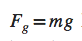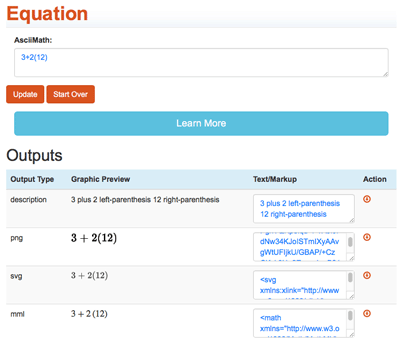Mathematics

Traditionally, math is challenging to render and to make accessible - thankfully in EPUB, there are techniques we can employ to provide multiple ways of perceiving and understanding math notation.

To encode math and math notations, we will be using MathML 3.0 which is a W3C specification (Link: W3C Math Home) that is widely supported by modern-day EPUB reader systems. To see how MathML works, let's use this physics formula for the force of gravity as an example: f<sub>g</sub> = mg.

<math xmlns="http://www.w3.org/1998/Math/MathML">
<mrow>
<msub>
<mi>f</mi>
<mi>g</mi>
</msub>
<mo>=</mo>
<mi>m</mi>
<mi>g</mi>
</mrow>
</math>

When viewed in an EPUB the formula looks great as seen in the following image:However, for someone using a screen reader or another accessibility tool, the formula may appear or sound like "f g equals m g" which is ambiguous. Is that "f g as in f times g" or is it "fg a variable"? To clear up any misunderstanding, it is recommended that a literal description be added using the aria-label property. It is important to not interpret or impart meaning to the description as this may cause some confusion - be as literal as possible and add just enough information to remove ambiguity.

In our example fg = mg example, a good literal description would be: "f subscript g equals m times g".

<math xmlns="http://www.w3.org/1998/Math/MathML"
aria-label="f subscript g equals m times g">

<mrow>
<msub>
<mi>f</mi>
<mi>g</mi>
</msub>
<mo>=</mo>
<mi>m</mi>
<mi>g</mi>
</mrow>
</math>

This is a good start, however someone may not be familiar with the subject matter or have cognitive considerations - this may require some additional details as a literal description alone lacks any context or elaboration.

Using the aria-describedby property, we can link math formulas and equations to a more detailed explanation.

<math xmlns="http://www.w3.org/1998/Math/MathML"
aria-label="f subscript g equals m times g"

<mrow>
<msub>
<mi>f</mi>
<mi>g</mi>
</msub>
<mo>=</mo>
<mi>m</mi>
<mi>g</mi>
</mrow>
</math>

The force of gravity acting on an object can be
calculated by multiplying the mass of the
object and the gravitational constant.
</p>

To ensure that standard compliant reader systems understand your math content, we wrap the [itex][/itex] content in a container (a <span>, <div>, or <p>) and give it a math role.

<span role="math">
<math xmlns="http://www.w3.org/1998/Math/MathML"
aria-label="f subscript g equals m times g"

<mrow>
<msub>
<mi>f</mi>
<mi>g</mi>
</msub>
<mo>=</mo>
<mi>m</mi>
<mi>g</mi>
</mrow>
</math>
</span>
The force of gravity acting on an object can be
calculated by multiplying the mass of the
object and the gravitational constant.
</p>

Units, Measurements, and Acronyms

Units and measurements poses their own unique challenge as units are often written in abbreviated form. For example, the letter "m" may mean "mass" or "metres" - both which may appear simultaneously in the content. To remove any ambiguity, the following technique would help clarify the intended meaning:

• For units within sections of MathML [itex] [/itex], use <mn mathvariant='normal' class='epub-mathML-Unit'>kg</mn>, mathvariant "normal" ensures that the unit notation does not render the same way as a number, making it distinct. epub-mathML-Unit is an example optional class you can use for styling.
• For units within rest of content and outside of [itex] blocks, use <abbr> for unit abbreviations with WAI-ARIA aria-label values. For example:
• <abbr role="text" title="kilograms" aria-label="kilograms">kg</abbr>
• <abbr role="text" title="meters per second" aria-label="meters per second">m/s</abbr>

MathML Cloud

MathML Cloud is a tool which converts math notation into readable text and other formats. MathML Cloud can take math notation in ASCII text, MathML or Latex notation, and output a text equivalent, PNG, SVG, and MML which can be embedded into EPUB 3 and HTML.The image shows the MathML interface converting an equation into different formats. Try MathMLCloud at https://www.mathmlcloud.org/

Related: MathML Cloud Project Description